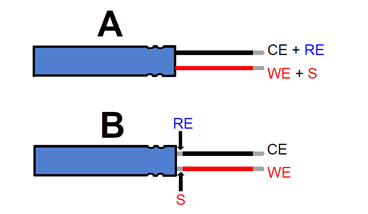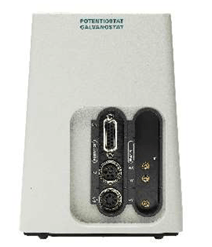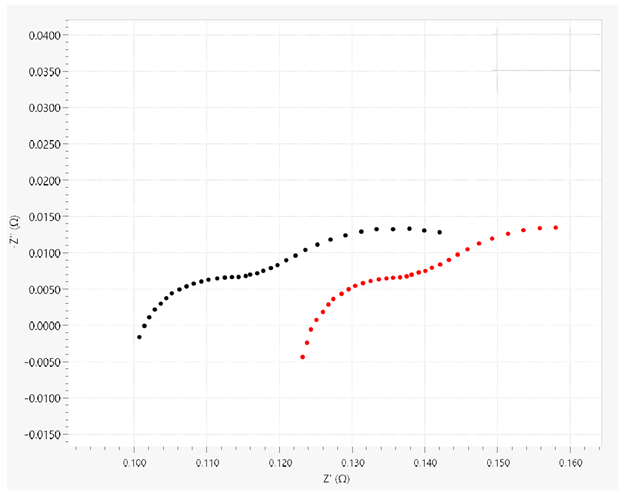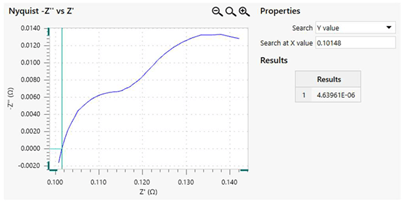# Electrochemical Impedance Spectroscopy Measurements: The Importance of Four-Terminal Sensing

Electrochemical impedance spectroscopy (EIS) is used in this procedure to test the impedance in a commercial battery after connecting it two ways. An analysis of the differences in the obtained values for impedance is also made.

Commercial batteries come under the label of low-impedance devices under test (DUTs). To find impedance in such devices, best practices have been set forth. The first one is to achieve separation between the leads that sense the potential difference and the leads that carry current.

The next one is to connect the potential-sensing leads with as little space between them and the DUT as possible, to minimize any extra wire which could artificially increase the impedance significantly in the case of such a device.

## First measurement of EIS

The battery impedance is sensed after it is connected through two terminals, with the RE and CE leads connected so as to short-circuit them, and both joined to the black wire which leads from the negative battery terminal. The WE and S wires are similarly joined together and also attached to the anode wire which is red.

## Second measurement of EIS

The battery sensing is done after connecting it through four terminals, also called a Kelvin sensing configuration. The CE wire and WE wire go to the cathode and anode respectively, the RE lead is attached to the meeting point of the battery and the cathode, and the S lead to the corresponding point between the anode and battery.Figure 1. Illustration of the two-terminal (A) and the four-terminal (B) sensing configurations.

The variation in the configuration of the leads will cause different impedance values to arise on measurement. This is because the RE and S leads are now measuring the potential difference between VRE-S, but the CE and WE leads are conducting current iCE-WE to combat the lowering of potential across the battery, namely, Vdrop.

The current value remains unchanged in both steps as there is no change in the configuration of the CE and WE wires. The potential drop is also constant, being calculated by the following equation:

 Vdrop = iCE-WE.Ztot = iCE-WE.(Zbat + Zwires) (1)

Ztot which represents the total impedance is the sum of the impedance of the battery and the wires, Zbat and Zwires. The potential difference VRE-S measured in the two-terminal configuration is still different from that in the Kelvin configuration. The RE and S is the measure of the potential difference VRE-S which can be calculated by multiplying  iCE-WE with (Zbat + Zwires). In short, VRE-S is the same as the Vdrop in equation 1.

 V(RE-S,A)=Vdrop = iCE-WE.(Zbat + Zwires) (2)

In the second configuration, the RE and S measure the potential difference which is equal to the product of iCE-WE and Zbat.

 VRE-S,B = iCE-WE.(Zbat) (3)

This, is why a two-terminal sensing configuration for EIS on a DUT will show higher values of impedance compared to the same test using a four-terminal configuration, and the difference is equal to Zwires.

## Experimental Setup

The EIS testing is performed using a Metrohm Autolab PGSTAT204 with a FRA32M module. In this experiment a rechargeable Li-ion Ansmann 18650 battery is used, with nominal capacity and nominal voltage of 2600 mAh and 3.7 V respectively.Figure 2. The Metrohm Autolab PGSTAT204, equipped with the FRA32M module.

The potentiostat measurements of EIS are carried out at open circuit potential (OCP) at frequencies between 1 kHz and 100 mHz, with an amplitude of 10 mV and a rate of 10 frequencies per decade.

## Results and Discussion

The EIS measurements using four-terminal and two-terminal configurations on the battery are plotted on Nyquist plots, showing the former to be generally lower than the latter.Figure 3. Nyquist plots from EIS measurements performed on the Li-ion battery with four-terminal (black dots) and two-terminal (red dots) sensing configurations.

The amplitude of the shift between the two plots is proportional to the impedance of the wires used in the two-terminal setup. This is estimated using the impedance values at -Z"=0 at which point the only contribution of impedance is resistive.

This is subtracted from the former to find the resistive component (due to the wires) in the overall impedance. Such results can be quickly obtained using the Interpolate command in the NOVA software.Figure 4. The Interpolate command on the Nyquist plot related to the four-terminal sensing EIS measurement.

Table 1. Values of the resistive contribution to the overall impedance for the two configurations

Connections R (mΩ)
Two-terminal sensing 124
Four-terminal sensing 101

If the difference in resistance values is found, it gives the resistive contribution of the leads in the two-terminal setup, or Rwires= 23 mΩ. Also, the latter configuration, when plotted, tails off towards the negative side of the imaginary or negative side of the impedance (-Z") more strongly as the frequency increases, a feature usually due to the inductance of the leads used, though this cannot now be explained in detail.

Another finding is that the Kelvin configuration is useful only when EIS is measured for low-impedance DUTs when the relatively small component of impedance due to the wires becomes important.

## Conclusions

Two sensing configurations were employed to measure the EIS of a commercial battery. The contribution of the four-terminal configuration in accurate EIS measurement of a low-impedance DUT is explored in the light of the results. The component of impedance due to the resistive characteristic of the wires and its importance in two-terminal sensing configurations in such a context is also calculated and discussed.This information has been sourced, reviewed and adapted from materials provided by Metrohm AG.

## Citations

• APA

Metrohm AG. (2020, May 01). Electrochemical Impedance Spectroscopy Measurements: The Importance of Four-Terminal Sensing. AZoM. Retrieved on October 27, 2020 from https://www.azom.com/article.aspx?ArticleID=15449.

• MLA

Metrohm AG. "Electrochemical Impedance Spectroscopy Measurements: The Importance of Four-Terminal Sensing". AZoM. 27 October 2020. <https://www.azom.com/article.aspx?ArticleID=15449>.

• Chicago

Metrohm AG. "Electrochemical Impedance Spectroscopy Measurements: The Importance of Four-Terminal Sensing". AZoM. https://www.azom.com/article.aspx?ArticleID=15449. (accessed October 27, 2020).

• Harvard

Metrohm AG. 2020. Electrochemical Impedance Spectroscopy Measurements: The Importance of Four-Terminal Sensing. AZoM, viewed 27 October 2020, https://www.azom.com/article.aspx?ArticleID=15449.

## Tell Us What You Think